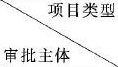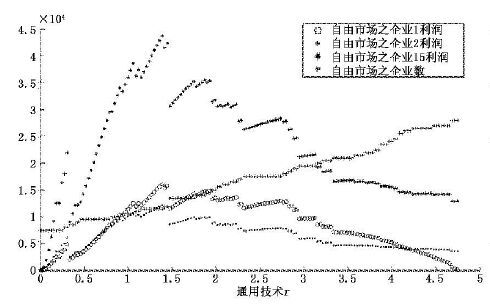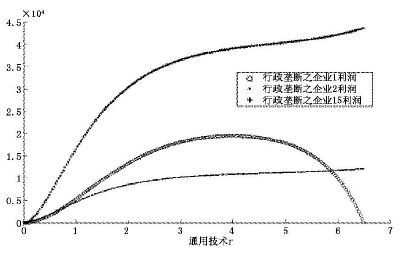﻿ 中国垄断性行业的准入壁垒及其经济绩效
 财经研究2016, Vol. 42Issue (6): 87-970

#### 文章信息

Chen Lin.

Entry Barriers and Economic Performance of Chinese Monopoly Industries

Journal of Finance and Economics, 2016, 42(6): 87-97.

### 文章历史

1.暨南大学 产业经济研究院产业组织与规制研究所，广东 广州 510632;
2.广州市自由贸易试验区研究基地，广东 广州 510632

Entry Barriers and Economic Performance of Chinese Monopoly Industries
Chen Lin1,2
1.Institute of Industrial Economics, Jinan University, Guangzhou 510632;
2.China;Institute of Guangzhou Free Trade Zone, Guangzhou 510632, China
Abstract: How to understand the important significance of administrative approval system reform of market access since the 18th CPC National Congress has become the hot topic in current theoretical realm. Different from previous empirical research, this paper models the administrative approval system by employing a mainstream economic model, i.e. dynamic competition innovation model, for the first time, and deeply analyzes the specific economic performance as well as its function mechanism. It comes to the main conclusions as follows:firstly, the life cycles of monopoly industries are longer than the ones of competitive industries, so the elimination of outdated capacity in monopoly industries is a relatively effective industrial policy if the monopoly cannot be abolished; secondly, monopoly industries give rise to much higher prices, and benefit the incumbent firms greatly by bringing more economic profits to them, so price and profit regulation are two essential tools for governments to manage monopoly industries; thirdly, since administrative entry barriers would extrude consumer surplus, the administration supervision departments should impose extra welfare tax on enterprises' monopoly rents, and build a policy system about transfer payments to consumers; fourthly, it is also necessary for supervision departments to implement innovation incentives since the existence of monopoly industries can inhibit technological progress inside the industries.
Key words: monopoly industry    administrative entry barrier    administrative approval    economic system reform非政府性资金,包括国民经济的全部行业,实施“备案制” 非政府性资金,《政府核准的投资项目目录》内的领域实施“核准制” 使用政府资金的企业投资项目实施“审批制” 国家发改委 - 额定规模以上的水利、电力、煤炭、石油、天然气、水陆运输、有(黑)色金属、石油化工、化肥、汽车、铁道车辆、船舶、纸浆、制糖、供水等;不分规模的电信、邮政、部分信息产业、民航、燃料乙醇、烟草、制盐、航空航天、城市轨道、文教金融等 使用中央财政资金项目 省级发改委 总投资2亿元及以上的基本建设投资项目 农业、水泥、年产十万吨以下的纸浆、制糖、投资五亿以下的矿山、储量五千万吨以下的铁矿、一百公里以下的省内铁道、五亿立方米以下的输气管网等 使用省级财政资金项目 市级发改部门 总投资3 000万元(含)-2亿元(不含)的基本建设投资项目 除法定由国家及省级发改委审批之外的核准项目 使用市级财政资金项目 县(市、区)发改部门 总投资3 000万元(不含)以下的基本建设投资项目 使用县(市、区)级财政资金项目 行政审批的审查依据 是否符合产业政策、行业准入标准、其他法律法规等 是否符合国家法律法规、经济社会规划、行业规划、产业政策、行业准入标准、土地利用总体规划、宏观调控政策、经济安全、生态环境和公众利益等 注:除工业、交通、商业领域技术改造投资项目由各级经贸部门备案外,其余领域技术改造项目由各级发改部门(发改部门为各级发展和改革委员会的简称)备案;除外资企业外,本表适用于国有、民营和集体企业;备案制的项目分级采用广东省的法定标准,各省略有不同。实施"备案制"的行业,发改部门一般采取自由裁量执法来对产业投资进行干预。资料来源为:作者根据相关法律法规、政策文件整理所得,由于涉及法条甚多,表 1无法一一罗列;备案制项目分级标准(投资金额)采用广东省标准,但实际上各省市之间略有差异。

 $U = a\left( {1 - {e^{ - r}}} \right){Q_r} - {Q_r}^2/2$ (1)

 ${P_r} = a\left( {1 - {e^{ - r}}} \right) - {Q_r}$ (2)

U代表消费者的效用函数,Qr≥0代表消费者的总消费量,a>0代表保留效用(a>0说明保留效用非负),Pr>0代表同质品市场的价格,e代表以其为底的对数(比如lnA=logeA)。r>0代表创新的水平。用反需求函数(2)对r求导,得:

 $d{P_r}/{\rm{dr = }}a{e^{ - r}} = a - {Q_r} - {p_r}$ (3)

r所代表的创新水平固定时,有dU/dQr=Pr>0、d2U/dQr2=dPr/dQr=-1<0,说明消费者的效用函数U随着总消费量Qr的递增而递增,但是递增的幅度却在逐渐减小;当Qr所代表的总消费量固定时,有dU/dr=ae-rQr≥0、d2U/dr2=-ae-rQr≤0,则消费者效用U随产品创新r同向变化,但变化的幅度也在递减。

 \eqalign{ &{\rm{MAX}}{P_r}{q_{i,r}} - \left( {{c_i} + {e^{ - {x_{i,r}}}}} \right){q_{i,r}}^2 - {x_{i,r}} \cr &{\rm{s}}{\rm{.t}}{q_{i,r}} \ge 0,{x_{i,r}} \ge 0 \cr} (4)

 ${\rm{MAX}}\int\limits_O^T {\left[{{P_r}{q_{1,r}} - \left( {{c_1} - {e^{ - r}}} \right){q_{2,r}}^2 - r} \right]} {\rm{d}}r$ (5)
 ${\rm{s}}{\rm{.td}}{P_r}/{\rm{dr = }}a{e^{ - r}} = a - {Q_r} - {p_r},{P_0} = 0,{Q_0} = {q_{1,0}} = 0$

q1,r是企业1的产量。r≥0是其创新投入,即整个产业的创新技术。为便于使用求解动态最优化的最优控制理论求解,假设远期收益与当期收益对企业产生的效用一致。根据目标函数(5)可知,为了求总收益的最优动态路径来实现长期收益的最大化,企业1的创新技术r必须位于［0,T］的增区间内。假设当r上升至T0时,企业1的利润m1,T0=PT0q1,T0-(c1+e-T0)q21,T0-T0＜0,企业1的利润为负,那么企业1的长期收益开始出现负增长,作为理性的企业1来说,此时退出市场将是最明智的选择。技术领导企业1可以最大化本身的长期利益,从而目标函数(5)得以实现,而技术模仿企业却只能最大化当期的利益,从而目标函数(4)得以实现。

 \eqalign{ &{\Pr _r}\left( {\Delta {n_r} = 0|\mathop {\lim }\limits_{\varepsilon \to {0^ + }} P'\_\left( {r - \varepsilon } \right) ＞ 0} \right) = {\Pr _r}\left( {\Delta {n_r} ＜ 1|\mathop {\lim }\limits_{\varepsilon \to {0^ + }} P'\_\left( {r - \varepsilon } \right) ＞ 0} \right) \cr & = \int\limits_{ - \infty }^1 {{1 \over {\sqrt {2\pi } }}} {{\rm{e}}^{{{ - \left( {t - \mu } \right)2} \over {2\sigma 2}}}}{\rm{d}}t \cr} (6)
 ${\Pr _r}\left( {\Delta {n_r} = m|\mathop {\lim }\limits_{\varepsilon \to {0^ + }} P'\_\left( {r - \varepsilon } \right) > 0} \right) = \int_m^{m + 1} {{1 \over {\sqrt {2\pi } }}{{\rm{e}}^{{{ - \left( {t - \mu } \right)2} \over {2\sigma 2}}}}{\rm{d}}t}$ (7)

 \eqalign{ &{\Pr _r}\left( {\Delta {n_r} = 0|{{\lim }_{\varepsilon \to {0^ + }}}P'\_\left( {r - \varepsilon } \right) \le 0} \right) = {\Pr _r}\left( {\Delta {n_r} ＜ 1|\mathop {\lim }\limits_{\varepsilon \to {0^ + }} P'\_\left( {r - \varepsilon } \right) \le 0} \right) \cr & = \int_{ - \infty }^1 {{1 \over {\sqrt {2\pi } }}} {{\rm{e}}^{{{ - \left( {t - \mu } \right)2} \over {2\sigma 2}}}}{\rm{d}}t \cr} (8)
 ${\Pr _r}\left( {\Delta {n_r} = m|{{\lim }_{\varepsilon \to {0^ + }}}P'\_\left( {r - \varepsilon } \right) \le 0} \right) = \int_m^{m + 1} {{1 \over {\sqrt {2\pi } }}{{\rm{e}}^{{{ - \left( {t - \mu } \right)2} \over {2\sigma 2}}}}{\rm{d}}t}$ (9)

 ${m_{i,r}} ＜ 0 或 {q_{i,r}} \le 1,\mathop {\lim }\limits_{\varepsilon \to {0^ + }} {q_{i,r + \varepsilon }} = 0$ (10)

mi,r,qi,r分别表示在创新技术为r时企业i的当期利润和产量。mi,r＜0意味着当当期利润小于零,qi,r≤1意味着当产量小于1的时候limε→0+qi,r+e=0,即表示企业i将不会再生产,企业的最优创新投入将出现非正值,此时企业i将会选择退出市场。

 ${R_1}\left( {{Q_{ - 1,r}},r,n} \right) = q_{1,r}^* = \left( {a - a{e^{ - r}} - {e^r} - {Q_{ - 1,r}}} \right)/\left( {2 + 2{c_1} + 2{e^{ - r}}} \right)$ (11)

2H(r,Pr,q1,r)/∂q21,r=-1-2c1-2e-r＜0,满足最优化二阶条件,且极大值唯一。联立并求解由式(3)和式(4)推导出来的一阶条件最优反应函数组成的微分方程组,便可获得动态博弈的纳什均衡解。联立一阶条件:∂(Prqi,r-(ci+e-xi,r)q2i,r-xi,r)/∂xi,r= e-xi,rq2i,r-1 =0和∂(Prqi,r-(ci+e-xi,r))q2i,r-xi,r)∂qi,r=Pr-2(ci+e-xi,r)qi,r=0,得企业i创新的最优反应函数式(12)和产量的最优反应函数式(13):

 $x_{i,r}^* = 1{\rm{n}}(q_{i,r}^{{*^2}})$ (12)
 ${R_i}({Q_{ - i,r}},r,n):q_{i,r}^* = a - a{e^{ - r}} - {Q_{ - i,r}} - \left( {1 + 2{c_i}} \right)q_{i,r}^* - 2/q_{i,r}^*$ (13)图 1 自由竞争市场之企业利润演化路径图图 2 行政垄断之企业利润演化路径图

(二) 垄断性行业的价格规制与利润规制

1. 价格规制

2. 利润规制

3. 转移支付

(三) 垄断性行业的创新绩效图 3 创新总量的演化路径图

  陈昆亭, 龚六堂. 粘滞价格模型以及对中国经济的数值模拟——对基本RBC模型的改进[J].数量经济技术经济研究,2006(8):106–117.  陈林, 朱卫平. 创新、市场结构与行政进入壁垒——基于中国工业企业数据的熊彼特假说实证检验[J].经济学(季刊),2011(2):653–674.  陈林, 罗莉娅, 康妮. 行政垄断与要素价格扭曲——基于中国工业全行业数据与内生性视角的实证检验[J].中国工业经济,2016(1):52–66.  陈林. 自由贸易区建设中的经验、误区与对策[J].经济学家,2016(5):5–13.  陈学云, 江可申. 航空运输业规制放松与反行政垄断——基于自然垄断的强度分析[J].中国工业经济,2008(6):67–76.  丁启军. 行政垄断行业高利润来源研究——高效率, 还是垄断定价?[J].产业经济研究,2010(5):36–43.  韩剑, 郑秋玲. 政府干预如何导致地区资源错配——基于行业内和行业间错配的分解[J].中国工业经济,2014(11):69–81.  靳来群, 林金忠, 丁诗诗. 行政垄断对所有制差异所致资源错配的影响[J].中国工业经济,2015(4):31–43.  林忠晶, 龚六堂. 退休年龄、教育年限与社会保障[J].经济学(季刊),2007(1):211–230.  罗党论, 刘晓龙. 政治关系、进入壁垒与企业绩效——来自中国民营上市公司的经验证据[J].管理世界,2009(5):97–106.  裴长洪. 经济新常态下中国扩大开放的绩效评价[J].经济研究,2015(4):4–20.  王俊豪, 王建明. 中国垄断性产业的行政垄断及其管制政策[J].中国工业经济,2007(12):30–37.  吴利学. 中国能源效率波动:理论解释、数值模拟及政策含义[J].经济研究,2009(5):130–142.  严海宁, 汪红梅. 国有企业利润来源解析:行政垄断抑或技术创新[J].改革,2009(11):128–133.  杨蹇, 刘华军. 中国烟草产业行政垄断及其绩效的实证研究[J].中国工业经济,2009(4):51–61.  于良春, 张伟. 中国行业性行政垄断的强度和效率损失研究[J].经济研究,2010(3):16–27.  周其仁.竞争、垄断和管制——"反垄断"政策的背景报告[A].王晓晔.产权与制度变迁[C].北京:北京大学出版社, 2004.  张伟, 于良春. 行业行政垄断的形成及治理机制研究[J].中国工业经济,2011(1):69–78.  Gordon R H, Li W. Government as a discriminating monopolist in the financial market[J].Journal of Public Economics,2003,87(2): 283–312.  Laffont J J. Regulation and development[M]. Cambridge: Cambridge University Press, 2000 .  Le T T. Reforming China's airline industry:From state-owned monopoly to market dynamism[J].Transportation Journal,1997,37(2): 45–62.